Thursday 21st January 2021

CBSE Guess > Papers > Question Papers > Class XII > 2004 > Chemistry > Compartment Delhi Set-I

CHEMISTRY (Set I—Compartmet Delhi)

Q. 1. Why is common salt sometimes yellow instead of being pure white? 1

Q. 2. What happens when blood cells are placed in pure water? 1

Q. 3. For the reaction 2X --> X2 , the rate of reaction becomes three times when the concentration of X is increased 27 times. What is the order of the reaction? 1

Q. 4. Write IUPAC name ofQ. 5. Give chemical test to distinguish between
C6H5CH2. NH2 and C6H5NH2

Q. 6. When would the wavelength associated with an electron be equal to the wave length associated with a proton?
Mass of electron = 9.1095 x 10-28 g; Mass of proton = 1.6725 x 10-24 g. 2

Q. 7. The equilibrium constant at 25° C for the processis 2.5 x 10 6 . Calculate the value of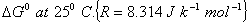.
In which direction is the reaction spontaneous under standard conditions? 2

Q. 8. Explain why electrolysis of aqueous solution of NaCI gives H2 at cathode and Cl2 at anode. Write overall reaction. 2

Q. 9. Write balanced chemical equations for the following reactions: 2

1. XeF2 + H2O -->
2. XeF4 + SbF5 -->
3. U + CIF3 -->
4. Ca3P2 + H2O -->

Q. 10. Name the alkene with least number of carbon atoms capable of exhibiting Chirality. Draw the structures of the possible enantiomers. 2

Or

How are enantiomers different from diastereomers? Give one example of each. 2

Q. 11. How are the following conversions carried out? 2

1. Ethanol to 1, 2 Ethane-diol
2. Phenol to Acetophenone

Q. 12. What is the difference between thermosetting and thermoplastic polymers? Give one example each. 2

Q. 13. Give reasons for the following: 3

1. When 30 ml of ethanol and 30 ml of water are mixed, the volume of resulting solution is more than 60 ml.
2.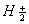and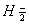ions have same bond order but H ions are more stable than.
3. Copper is conducting as such, whereas copper sulphate is conducting only in aqueous solution.

Q. 14. What is a semiconductor? Describe the two main types of semiconductors and contrast conduction mechanism in them. 3

Q. 15. 2 g of C6H5COOh dissolved in 25 g of benzene shows a depression in freezing point equal to 1.62 K. Molar depression constant for benzene is 4.9 K kg mol-1 is the percentage association of acid if it exists as dimers in solution? 3

Q. 16. What is meant by entropy? Predict the sign of entropy change in each of the following: 3

1.2.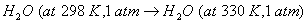3.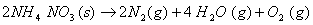4. Crystallization of copper sulphate from its saturated solution.
5.Q. 17. When a certain conductivity cell was filled with 0.1 M KCI, it has a resistance ofat 25° C. When the same cell was filled with an aqueous solution of 0.052 M unknown electrolyte, the resistance was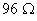. Calculate the molar conductivity of the unknown electrolyte at this concentration.
(Specific conductivity of 0.1 M KCI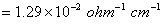) 3

Q. 18. (a) How is half-life period related to Initial concentration for a second order reaction?
(b) Dissociation of SO2 CI2 in the gas phase is a first order reaction with rate constant 2.3 x 10-5 s-1 at 600 K. Calculate the percentage of SO2 CI2 that would remain after 200 minutes of reaction time. 3

Q. 19. Explain what is observed when

1. A beam of light is passed through a colloidal solution of As2S3 .
2. An electrolyte (NaCI) is added to ferric hydroxide sot.
3. An electric current is passed through a colloidal solution. 3

Q. 20. (a) Write the formula of the following complexes:

1. Hexaammineplatinum (IV) chloride
2. Dichlorotetraamminecobalt (III) ion
3. Using valence bond theory of complexes, explain the geometry and magnetic nature of 3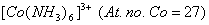Or

(a) The values of dissociation constant of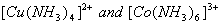are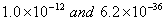respectively. Which complex would be more stable and why?

(b) Using valence bond theory of complexes, explain the geometry and mag netic nature of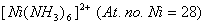3

Q. 21. (a) If an atomic nucleus does not contain electrons, then how can it emit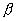particles?
(b) Which of the two, fusion or fission reactions, is more useful at present for peaceful purposes and why?
(c) State what is essentially a nuclear reactor. 3

Q. 22. Give reasons for the following facts:

1. Aldehydes are more reactive than ketones towards nucleophilic reactions.
2. Chloroacetic acid is stronger than acetic acid.
3. Treatment of benzaldehyde with HCN gives a mixture of two isomers which cannot be separated even by careful fractional distillation.

Q. 23. (a) Explain the following giving suitable examples:

1. Coupling reaction
2. Sandmeyer reaction
3. Give a chemical test to distinguish between 1-nitropropane and 2-nitropropane. 3

Q. 24. Define the following and give one example of each: 3

1. Tranquillizers
2. Mordant
3. Hybrid rocket propellant

Q. 25. Give reasons for each of the following: 5

1. Bleaching of flowers by Cl2 is permanent while by SO2 is temporary.
2. Molten aluminium bromide is a poor conductor of electricity.
3. Nitric oxide becomes brown when released in air.
4. PCl5 ionic in the solid state.
5. Ammonia is a good complexing agent.

Or

Name the principal ores of tin and lead and describe in brief how these metals are extracted from their respective ores. 5

Q. 26. (a) Explain the following:

1. Transition elements tend to be unreactive with increasing atomic number in the series.
2. d-block elements exhibit more oxidation states than f-block elements.

(b) A green chromium compound (A) on fusion with alkali gives a yellow com pound (B) which on acidification gives an orange coloured compound (C). 'C' on treatment with NH4 CI gives an orange coloured product (D), which on heating decomposes to give back (A). Identify A, B, C and D. Write equations for reactions. 2, 3

Q. 27. (a) Describe the following giving one example each:

1. Co-enzymes
2. Mutation in biomolecules
3. Nucleotides
4. List four functions of carbohydrates in living organisms. 3, 2
 Chemistry 2004 Question Papers Class XII Delhi Outside Delhi Compartment Delhi Compartment Outside DelhiSet ISet ISet ISet ISet IISet IISet IISet IISet IIISet III

CBSE 2004 Question Papers Class XII